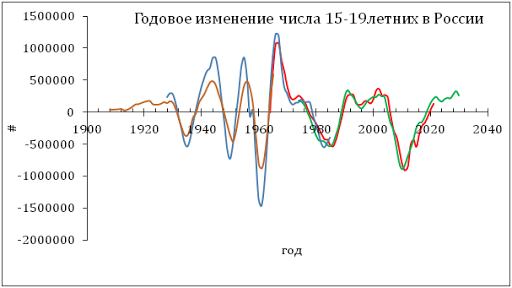## 1/20/12

### Quarterly report on Procter and Gamble

This is a quarterly update of the share price model for Procter and Gamble as based on the decomposition into a weighted sum of two consumer price indices (to be determined), linear time trend and constant. It is shown that the model is valid since September 2009 at least and does not show any sign of possible failure. It predicts the share price at a four month horizon.

A share price model for Procter and Gamble (NYSE: PG) was originally published in this blog in July 2010.  According to our concept, it was defined by the index of food away from home (SEFV - CUUS0000SEFV) and that of rent of primary residency (RPR); the evolution of these indices is presented in Figure 1. In the original model, the former CPI component led the share price by 3 months and the latter one led by 8 months. The upper panel of Figure 2 depicts the original model and the monthly closing prices available in July 2010. This original model was stable for the previous 11 months, i.e. for the period from September 2009.

In April 2011, we updated the original model using some new data (closing price for March 2011) and found that the same model was also applicable with a small change in the time lead for the SEFV – it was 4 months instead of 3 months in the original model.  New coefficients were also slightly different, but very close to the original ones.

The September’s update uses the monthly closing price for September 2011 and CPIs for August 2011. It validates the model obtained for the previous period but is characterized by the same time lags and a small shift in the coefficients estimated by the LSQ technique. The current update for the December 2011 closing price is obtained using the CPI data for December 2011 and thus both time shifts are one month longer. Four best-fit models for PG(t) are as follows:

PG(t) =  -5.88SEFV(t-3) + 3.43RPR(t-8)  + 17.60(t-1990) + 174.08, July 2010

PG(t) =  -5.40SEFV(t-4) + 2.93RPR(t-8)  + 18.16(t-1990) + 187.47, March 2011

PG(t) =  -4.94SEFV(t-4) + 2.47RPR(t-8)  + 18.15(t-1990) + 184.89, September 2011

PG(t) =  -4.76SEFV(t-5) + 2.27RPR(t-9)  + 18.29(t-1990) + 187.61, December 2011

where PG(t) is the monthly closing price (dividend and split adjusted) in U.S. dollars,  t is calendar time.

In the lowermost panel of Figure 2, the predicted curve leads the observed price by 5 months with the residual error of \$2.16 for the period between July 2003 and December 2011 (see Figure 3 for the model residuals). In other words, the price of a PG share is completely defined by the behaviour of these two CPI components.

The model does predict the share price in the past and foresaw a period of no growth in the fourth quarter of 2011. In January-March 2012, the price may fall.

Figure 1. Evolution of the price of SEFV and RPR.

Figure 2. Observed and predicted PG share prices as obtained using contemporaneous data

Figure 3. The model residual error.

1.In April 2011 tructiepbongda k+, we updated the original model using some new data (closing price for March 2011) and found that the same model was also applicable with a small change in the time lead for the SEFV – it was 4 months instead of 3 months in the original model bongdatructuyen. New coefficients were also slightly different, but very close to the original ones.

2.Observed chơi đá gà and predicted PG share prices as obtained using contemporaneous data trực tiếp đá gà cựa sắt

### История России в 20 веке в одной кривой изменения численности населения: Германия проиграла две войны, но, вероятно, убила СССР, убив его граждан

Реальный экономический рост и социальный прогресс в решающей степени зависят от притока нового населения трудоспособного возраста, то есть л...